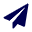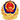• 全部 (4)
• 2020(4)####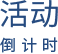var timestamp = Date.parse(new Date()); var date = 1599580800+"000"; var daojishi = parseInt(date - timestamp) / 1000 / 60 / 60 / 24; //两个时间相差的小时数 if (daojishi <= 0) { daojishi = 0; } daojishi = daojishi.toString(); daojishi = daojishi.split("."); //取小数点前面的数字 daojishi = daojishi; var html = ""; for (var i = 0; i < daojishi.length && i < 3; i++) { html += "<div class='shuzi'>" + daojishi.substr(i, 1) + "</div>"; } document.write(html);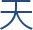####var timestamp = Date.parse(new Date()); var date = 1599062400+"000"; var daojishi = parseInt(date - timestamp) / 1000 / 60 / 60 / 24; //两个时间相差的小时数 if (daojishi <= 0) { daojishi = 0; } daojishi = daojishi.toString(); daojishi = daojishi.split("."); //取小数点前面的数字 daojishi = daojishi; var html = ""; for (var i = 0; i < daojishi.length && i < 3; i++) { html += "<div class='shuzi'>" + daojishi.substr(i, 1) + "</div>"; } document.write(html);####var timestamp = Date.parse(new Date()); var date = 1607529600+"000"; var daojishi = parseInt(date - timestamp) / 1000 / 60 / 60 / 24; //两个时间相差的小时数 if (daojishi <= 0) { daojishi = 0; } daojishi = daojishi.toString(); daojishi = daojishi.split("."); //取小数点前面的数字 daojishi = daojishi; var html = ""; for (var i = 0; i < daojishi.length && i < 3; i++) { html += "<div class='shuzi'>" + daojishi.substr(i, 1) + "</div>"; } document.write(html);####var timestamp = Date.parse(new Date()); var date = 1602604800+"000"; var daojishi = parseInt(date - timestamp) / 1000 / 60 / 60 / 24; //两个时间相差的小时数 if (daojishi <= 0) { daojishi = 0; } daojishi = daojishi.toString(); daojishi = daojishi.split("."); //取小数点前面的数字 daojishi = daojishi; var html = ""; for (var i = 0; i < daojishi.length && i < 3; i++) { html += "<div class='shuzi'>" + daojishi.substr(i, 1) + "</div>"; } document.write(html);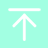### 获取最新资讯